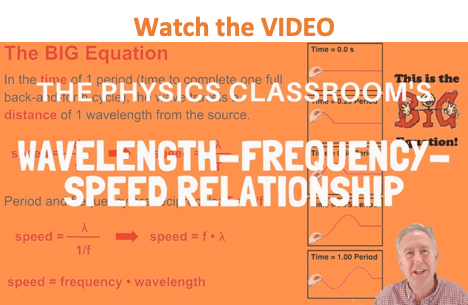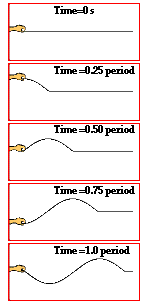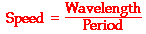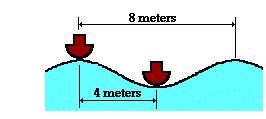Vibrations and Waves - Lesson 2 - Properties of a Wave

# The Wave EquationAs was discussed in Lesson 1, a wave is produced when a vibrating source periodically disturbs the first particle of a medium. This creates a wave pattern that begins to travel along the medium from particle to particle. The frequency at which each individual particle vibrates is equal to the frequency at which the source vibrates. Similarly, the period of vibration of each individual particle in the medium is equal to the period of vibration of the source. In one period, the source is able to displace the first particle upwards from rest, back to rest, downwards from rest, and finally back to rest. This complete back-and-forth movement constitutes one complete wave cycle.The diagrams at the right show several "snapshots" of the production of a wave within a rope. The motion of the disturbance along the medium after every one-fourth of a period is depicted. Observe that in the time it takes from the first to the last snapshot, the hand has made one complete back-and-forth motion. A period has elapsed. Observe that during this same amount of time, the leading edge of the disturbance has moved a distance equal to one complete wavelength. So in a time of one period, the wave has moved a distance of one wavelength. Combining this information with the equation for speed (speed = distance/time), it can be said that the speed of a wave is also the wavelength/period.Since the period is the reciprocal of the frequency, the expression 1/f can be substituted into the above equation for period. Rearranging the equation yields a new equation of the form:

Speed = Wavelength • Frequency

The above equation is known as the wave equation. It states the mathematical relationship between the speed (v) of a wave and its wavelength (λ) and frequency (f). Using the symbols v, λ, and f, the equation can be rewritten as

v = f • λ

As a test of your understanding of the wave equation and its mathematical use in analyzing wave motion, consider the following three-part question:

Stan and Anna are conducting a slinky experiment. They are studying the possible effect of several variables upon the speed of a wave in a slinky. Their data table is shown below. Fill in the blanks in the table, analyze the data, and answer the following questions.

 Medium Wavelength Frequency Speed Zinc, 1-in. dia. coils 1.75 m 2.0 Hz ______ Zinc, 1-in. dia. coils 0.90 m 3.9 Hz ______ Copper, 1-in. dia. coils 1.19 m 2.1 Hz ______ Copper, 1-in. dia. coils 0.60 m 4.2 Hz ______ Zinc, 3-in. dia. coils 0.95 m 2.2 Hz ______ Zinc, 3-in. dia. coils 1.82 m 1.2 Hz ______

1.  As the wavelength of a wave in a uniform medium increases, its speed will _____.

 a. decrease b. increase c. remain the same

2. As the wavelength of a wave in a uniform medium increases, its frequency will _____.

 a. decrease b. increase c. remain the same

3. The speed of a wave depends upon (i.e., is causally affected by) ...

a. the properties of the medium through which the wave travels

b. the wavelength of the wave.

c. the frequency of the wave.

d. both the wavelength and the frequency of the wave.

The above example illustrates how to use the wave equation to solve mathematical problems. It also illustrates the principle that wave speed is dependent upon medium properties and independent of wave properties. Even though the wave speed is calculated by multiplying wavelength by frequency, an alteration in wavelength does not affect wave speed. Rather, an alteration in wavelength affects the frequency in an inverse manner. A doubling of the wavelength results in a halving of the frequency; yet the wave speed is not changed.

1. Two waves on identical strings have frequencies in a ratio of 2 to 1. If their wave speeds are the same, then how do their wavelengths compare?

 a. 2:1 b. 1:2 c. 4:1 d. 1:4

2. Mac and Tosh stand 8 meters apart and demonstrate the motion of a transverse wave on a snakey. The wave e can be described as having a vertical distance of 32 cm from a trough to a crest, a frequency of 2.4 Hz, and a horizontal distance of 48 cm from a crest to the nearest trough. Determine the amplitude, period, and wavelength and speed of such a wave.

3. Dawn and Aram have stretched a slinky between them and begin experimenting with waves. As the frequency of the waves is doubled,

a. the wavelength is halved and the speed remains constant

b. the wavelength remains constant and the speed is doubled

c. both the wavelength and the speed are halved.

d. both the wavelength and the speed remain constant.

4. A ruby-throated hummingbird beats its wings at a rate of about 70 wing beats per second.

a. What is the frequency in Hertz of the sound wave?

b. Assuming the sound wave moves with a velocity of 350 m/s, what is the wavelength of the wave?

5. Ocean waves are observed to travel along the water surface during a developing storm. A Coast Guard weather station observes that there is a vertical distance from high point to low point of 4.6 meters and a horizontal distance of 8.6 meters between adjacent crests. The waves splash into the station once every 6.2 seconds. Determine the frequency and the speed of these waves.6. Two boats are anchored 4 meters apart. They bob up and down, returning to the same up position every 3 seconds. When one is up the other is down. There are never any wave crests between the boats. Calculate the speed of the waves.